﻿ 电动汽车的多点接入对区域电网的稳定性影响 The Impact of Electric Vehicles on the Stability of Regional Power Grids

Smart Grid
Vol.08 No.04(2018), Article ID:26410,12 pages
10.12677/SG.2018.84036

The Impact of Electric Vehicles on the Stability of Regional Power Grids

Di Luo1, Jingjing Wang2, Runqiu Li1, Yikun Jiao1, Jing Liu2, Ye Chen2

1State Grid Shaanxi Electric Power Economic Technology Research Institute, Xi’an Shaanxi

2School of Water Resources and Hydroelectric Engineering, Xi’an University of Technology, Xi’an Shaanxi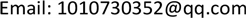Received: Jul. 14th, 2018; accepted: Jul. 28th, 2018; published: Aug. 14th, 2018ABSTRACT

This paper is based on the PSASP simulation system, using New England 10-machine 39-node network as the simulation network, analyzes the impact of the electric vehicle (EV) access mode on the system operating characteristics, and calculates the load, voltage offset, initial/limit point participation factor and line margin change under three access points, such as the three access points alone, two or two, and three buses in the power grid. The study found that when multiple buses are connected to an electric vehicle at the same time, the peak-to-valley load difference of the power grid can be reduced by 8.3%, the voltage offset rate can increase to 1.2205%, and the voltage initial point participation factor increases, while the limit point participation factor increases. With the reduction, the line stability margin can be reduced by a maximum of 3.33%. The above results show that the reasonable access of the electric vehicle has a deterrent effect on the daily load curve of the power system, and the weak bus of the power grid will change with the load change.

Keywords:Electric Vehicle Access, Access Mode, Stability, PSASP

1国网陕西省电力公司经济技术研究院，陕西 西安

2西安理工大学水利水电学院，陕西 西安1. 引言

2. 电动汽车入网模式分析

3. 静态电压稳定分析原理

3.1. 潮流方程式的特征结构分析

$\left[\begin{array}{l}\Delta P\\ \Delta Q\end{array}\right]={J}_{S}\left[\begin{array}{l}\Delta \theta \\ \Delta V\end{array}\right]$ (1)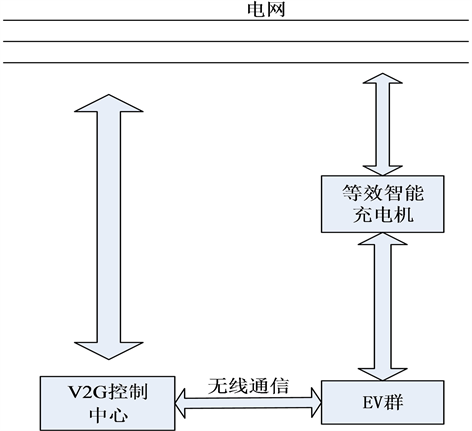Figure 1. Schematic of electric vehicles accessing to power grid

$\Delta Q=\left(L-J{M}^{-1}N\right)\Delta V={J}_{R}\Delta V$ (2)

${J}_{R}={\eta }^{-1}\Lambda \eta$ (3)

$\xi ={\eta }^{-1}$ ，则

$\Delta V=\sum _{i}\frac{{\xi }_{i}{\eta }_{i}}{{\lambda }_{i}}\Delta Q$ (4)

$\Delta V=\sum _{i}\frac{{\xi }_{i}{\eta }_{i}}{{\lambda }_{i}}\Delta Q$ (5)

$\eta \Delta V={\Lambda }^{-1}\eta \Delta Q$ (6)

$\eta \Delta V=\Delta \nu$$\eta \Delta Q=\Delta \rho$ ，则有

$\Delta \nu ={\Lambda }^{-1}\Delta \rho$ (7)

${\lambda }_{i}\Delta {\nu }_{i}=\Delta {\rho }_{i}$ (8)

3.2. 薄弱节点和薄弱区域的确定

$\frac{\partial {V}_{k}}{\partial {Q}_{k}}=\sum _{i}\frac{{\xi }_{ki}{\eta }_{ik}}{{\lambda }_{i}}$ (9)

${P}_{ki}={\xi }_{ki}{\eta }_{ik}$ (10)

4. 算例仿真

4.1. 算例系统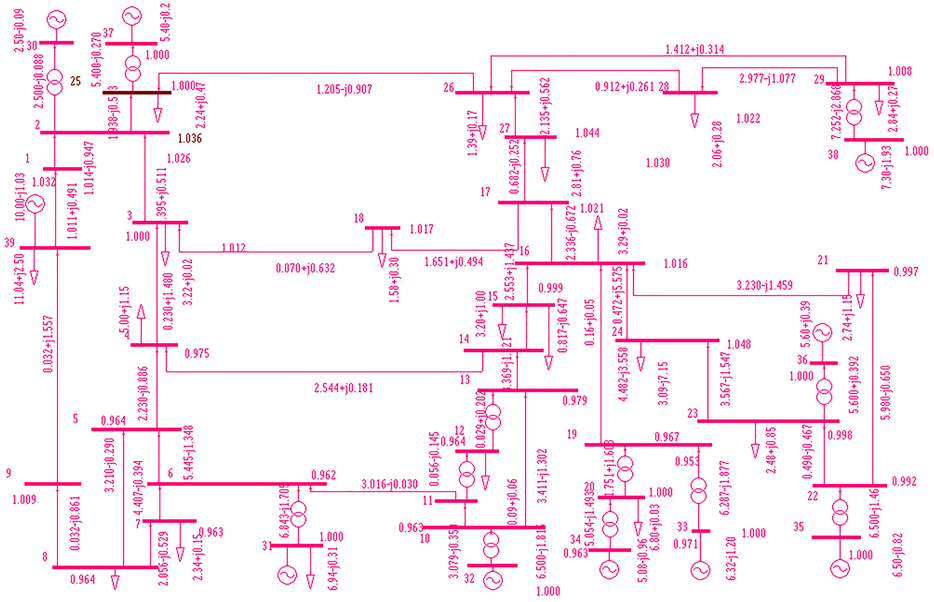Figure 2. Network flow of New England system

4.2. 仿真分析

4.2.1. 电动汽车接入对负荷的影响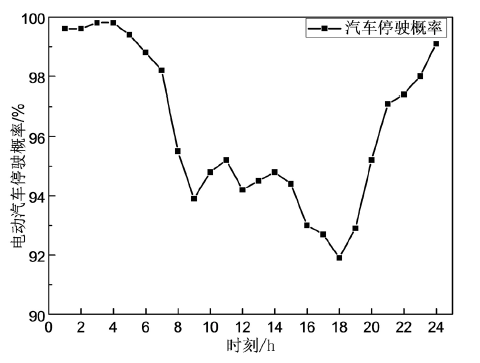Figure 3. Probability of a vehicle to be parkedFigure 4. The impact of electric vehicle access on power grid load

$\chi =\frac{\sigma }{\mu }$ (11)

4.2.2. 电动汽车入网对节点电压的影响

$\Delta U\left(%\right)=\frac{U-{U}_{ref}}{{U}_{ref}}×100%$ (12)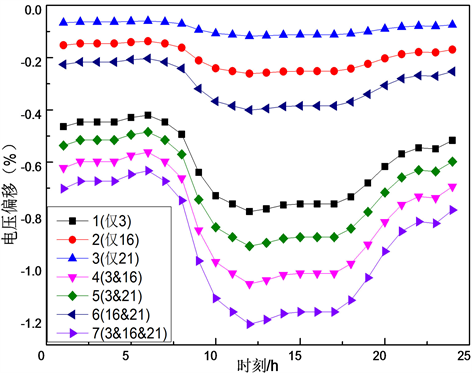Figure 5. Voltage excursion of different access conditions of electric vehicles

4.2.3. 电动汽车入网对母线参与因子的影响

PSASP以模态分析方法来寻找网络中的薄弱节点及区域，在初始运行点与电压稳定极限点处分别进行模态分析，进一步得出各节点对主导电压失稳的参与因子，由参与因子的大小来判断电网的薄弱节点区域。参与因子越大即此节点越薄弱。表3为初始/极限运行点处节点母线参与因子的计算结果  。

4.2.4. 电动汽车入网对线路裕度的影响Table 3. Results of bus participating factor for New England systemTable 4. Calculation results of line margin (%)

${K}_{L}=\frac{P-{P}_{0}}{{P}_{0}}$ (13)

5. 结论

1) 多母线接入电动汽车时，其对电网削峰填谷作用最为显著，其峰值降低了2.02%，谷值增加4.2%。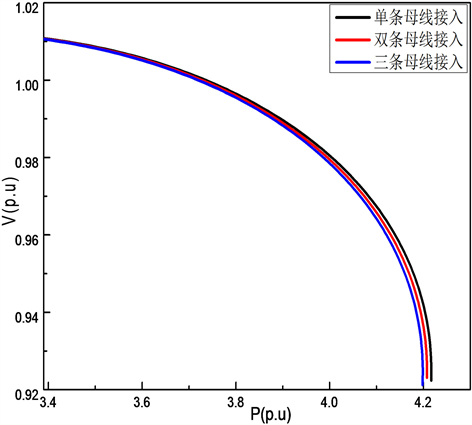Figure 6. P-V curve of busbar 3 under different access conditions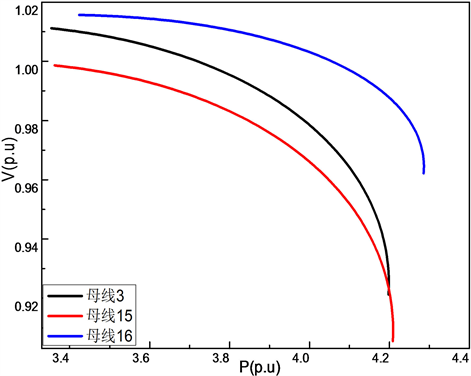Figure 7. P-V curves of different buses at three-point access

2) 参与因子的大小与母线承受扰动的能力呈现负相关的趋势，即参与因子越小，母线承受扰动的能力越强；三母共同接入模式下，参与因子的波动最大，这说明多点同时接入电动汽车时，各母线可承受的扰动相比最小。

3) 随着接入母线数量的增加，线路裕度逐步下降。三母线接入时，线路裕度降低可降低3.33%。

The Impact of Electric Vehicles on the Stability of Regional Power Grids[J]. 智能电网, 2018, 08(04): 323-334. https://doi.org/10.12677/SG.2018.84036

1. 1. 胡泽春, 宋永华, 徐智威, 等. 电动汽车接入电网的影响与利用[J]. 中国电机工程学报, 2012, 32(4): 1-10.

2. 2. Ungar, E. and Fell, K. (2010) Plug in, Turn on, and Load up. IEEE Power & Energy Magazine, 8, 30-35. https://doi.org/10.1109/MPE.2010.936354

3. 3. 李惠玲, 白晓民. 电动汽车充电对配电网的影响及对策[J]. 电力系统自动化, 2011, 35(17): 38-43.

4. 4. Singh, M., Kar, I. and Kumar, P. (2010) Influence of EV on Grid Power Quality and Optimizing the Charging Schedule to Mitigate Voltage Imbalance and Reduce Power Loss. 14th Interna-tional Power Electronics and Motion Control Conference, Orchid, 6-8 September 2010, 196-203. https://doi.org/10.1109/EPEPEMC.2010.5606657

5. 5. Soares, F.J., Peas Lopes, J.A. and Almeida, P.M.R. (2010) A Monte Carlo Method to Evaluate Electric Vehicles Impacts in Distribution Networks. IEEE Conference on Innovative Technologies for an Efficient and Reliable Electricity Supply (CITRES), Waltham, 27-29 September 2010, 365-372. https://doi.org/10.1109/CITRES.2010.5619777

6. 6. 郑颖, 孙近文, 张冲, 等. 考虑电动汽车接入的配电系统静态电压稳定裕度研究[J]. 电工技术学报, 2014, 29(8): 20-26.

7. 7. 甄浩庆, 王建全, 杨朝阳, 等. 通过电动汽车换电站提高电力系统暂态稳定性的研究[J]. 机电工程, 2014, 31(2): 229-233.

8. 8. Clement-Nyns, K., Haesen, E. and Driesen, J. (2010) The Impact of Charging Plug-In Hybrid Electric Vehicles on a Residential Distribution Grid. IEEE Transactions on Power Systems, 25, 371-380. https://doi.org/10.1109/TPWRS.2009.2036481

9. 9. Hadley, S.W. and Tsvetkova, A. (2008) Potential Impacts of Plug-In Hybrid Electric Vehicles of Regional Power Generation. Oak Ridge National Laboratory, U.S. Department of Energy, ORNL/TM-2007/1_50. https://doi.org/10.2172/932632

10. 10. Meyers, M.K., Schneider, K. and Pratt, R. (2007) Impacts Assessment of Plug-In Hybrid Vehicles on Electric Utilities and Regional U.S. Power Grids Part 1: Technical Analysis. Pacific Northwest National Laboratory, Washington DC.

11. 11. Scott, M.J., Meyers, M.K., Elliott, D.B., et al. (2007) Impacts Assessment of Plug-In Hybrid Vehicles on Electric Utilities and Regional U.S. Power Grids Part 2: Economic Assess-ment. Pacific Northwest National Laboratory, Washington DC.

12. 12. Lindly, J. and Haskew, T. (2002) Impact of Electric Vehicles on Electric Power Generation and Global Environmental Change. Advances in Environmental Research, 6, 291-302. https://doi.org/10.1016/S1093-0191(01)00060-0

13. 13. Snandez, L.P., Roman, T.G.S., Cossent, R., et al. (2011) Assessment of the Impact of Plug-In Electric Vehicles on Distribution Networks. IEEE Transactions on Power Systems, 26, 206-213. https://doi.org/10.1109/TPWRS.2010.2049133

14. 14. 王建. 电动汽车充电对电网的影响及有序充电研究[D]: [博士学位论文]. 济南: 山东大学, 2013.

15. 15. 邵尹池, 穆云飞, 余晓丹, 等. “车–路–网”模式下电动汽车充电负荷时空预测及其对配电网潮流的影响[J]. 中国电机工程学报, 2017, 37(18): 5207-5219.

16. 16. 葛少云, 郭建伟, 刘洪, 等. 计及需求侧响应及区域风光出力的电动汽车有序充电对电网负荷曲线的影响[J]. 电网技术, 2014, 98(7): 1806-1811.

17. 17. Zhao, L. and Aravinthan, V. (2013) Strategies of Residential Peak Shaving with Integration of Demand Response and V2H. Asia-Pacific Power and Energy Engineering Conference, Kowloon, 8-11 December 2013, 1-5.

18. 18. Shao, S., Manila, P. and Saifur, R. (2011) Demand Response as a Load Shaping Tool in an Intelligent Grid with Electric Vehicles. IEEE Transactions on Smart Grid, 2, 624-631. https://doi.org/10.1109/TSG.2011.2164583

19. 19. Shao, S., Manila, P. and Saifur, R. (2012) Grid Integration of Electric Vehicles and Demand Response with Customer Choice. IEEE Transactions on Smart Grid, 3, 543-550. https://doi.org/10.1109/TSG.2011.2164949

20. 20. 刘俊涛. 市场环境下计及参与需求侧响应的电动汽车换电站优化规划[J]. 华北电力大学学报, 2016, 43(2): 43-49.

21. 21. 权会霞, 刘宗歧. 基于代理模式的电动汽车参与调频服务PSASP建模[J]. 现代电力, 2013, 30(1): 66-70.

22. 22. PSASP电压稳定用户手册[Z]. 北京: 中国电力科学研究院, 2005.

23. 23. 张建华, 张国华, 杨京燕. 基于PSASP的廊坊电网静态电压稳定性分析[J]. 电力系统保护与控制, 2009, 37(5): 65-70.

24. 24. 王亦, 吴俊明, 翟云峰, 等. 考虑大规模风电和电动汽车入网的协同优化调度[J]. 电力科学与工程, 2015(4): 11-17.

25. 25. 罗航. 博弈论在含电动汽车区域电网优化调度中的应用[D]: [硕士学位论文]. 西安: 西安理工大学, 2017.

26. 26. 贺文武. 论电力负荷特性指数[J]. 长沙电力学院学报(自然科学版), 2004(4): 68-71.